# Magnetic Sector¶

A charged particle (mass m and charge q) with velocity v perpendicular to a uniform magnetic field B will (by the Lorentz Force Law) experience a centripetal force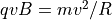, sustaining a circular motion of radius R in the plane perpendicular to the magnetic field   . This equation is called the cyclotron formula  , and it may be rewritten as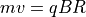, thereby showing that the gyroradius , R, is proportional to momentum, if charge is constant. A uniform magnetic field therefore can act as a momentum analyzer (in contrast to electric fields used in kinetic energy analyzers like in the Hemispherical Deflection Analyzer (HDA)).

A magnetic sector is a type of mass analyzer using a static magnetic field to deflect particles in this way along a roughly circular arc. It is characterized by a deflection angle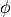and radius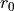. For background/theory, particularly on focusing properties in design, see   .

See also Hemispherical Deflection Analyzer (HDA), which is combined with a magnetic sector in a double focusing magnetic sector.

## SIMION specific¶

See SIMION Example: magnet (mag90.iob) for a simple 3D simulation with cylindrical poles and fringing fields. SIMION Example: magnetic_sector (added in 8.1.1.0) is a more extensive example, which examines focusing properties of various magnetic sector geometries (inclined and conical, 2D and 3D) with full control of parameters. The HIPIRMS course  includes other SIMION magnetic sector models and discussions. The Short ASMS Course (courses\short) (Session 2) has a brief look at sectors. SIMION simulations of sectors are illustrated in the discussions in . Magnetic poles with approximately infinite permeability and treated with scalar magnetic potential are the easiest to handle (see Magnetic Potential).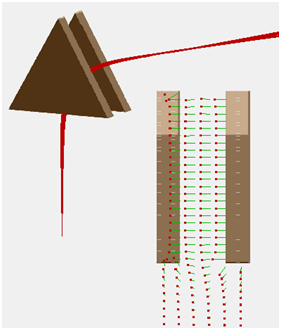Fig. 44 Figure: SIMION Example: magnetic_sector - 60 degree 3D sector (with fringe fields) and B-field vectors.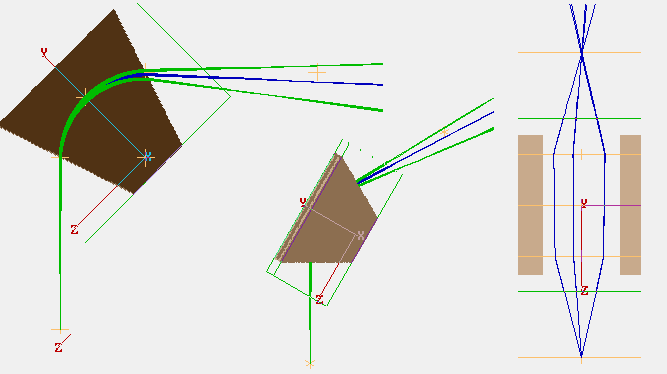Fig. 45 Figure: SIMION Example: magnetic_sector - 90 degree 3D sector (with fringe fields) and inclined 26.56 degree entrance/exit angles to achieve symmetric stigmatic focusing.